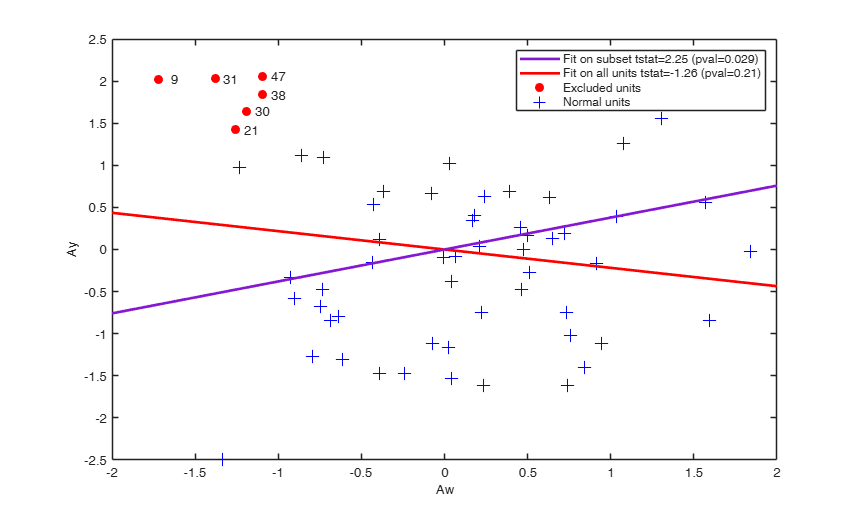# Documentation

## Syntax

• out=addt(y,X,w)example
• out=addt(y,X,w,Name,Value)example

## Description

 out =addt(y, X, w) addt with all default options.

 out =addt(y, X, w, Name, Value) addt with optional arguments.

## Examples

expand all

###addt with all default options.

Compute the t test for an additional regressor.

XX=load('wool.txt');
y=log(XX(:,end));
X=XX(:,1:end-2);
w=XX(:,end-1);
% out.Tadd (equal to -8.9707) is exactly equal to stats.tstat.t(4)
% obtained as
whichstats = {'tstat','mse'};
stats = regstats(y,XX(:,1:end-1),'linear',whichstats);
% Similarly out.S2add (equal to 0.0345) is exactly equal to stats.mse (estimate of
% \sigma^2 for augmented model)

###addt with optional arguments.

Excluding one observation from the sample; compare the added variable plot based on all units with that which excludes unit 43.

load('multiple_regression.txt');
y=multiple_regression(:,4);
X=multiple_regression(:,1:3);
[out]=addt(y,X(:,2:3),X(:,1),'plots',1,'units',,'textlab',true);## Related Examples

expand all

###Excluding more than one observation from the sample.

Compare the added variable plot based on all units with that which excludes units 9,21,30,31,38 and 47.

load('multiple_regression.txt');
y=multiple_regression(:,4);
X=multiple_regression(:,1:3);
[out]=addt(y,X(:,2:3),X(:,1),'plots',1,'units',[9 21 30 31 38 47]','textlab',true);## Input Arguments

### y — A vector with n elements that contains the response variable. y can be both a row of column vector.

Data Types: single| double

### X — Data matrix of explanatory variables (also called 'regressors'). Rows of X represent observations and columns represent variables.

Missing values (NaN's) and infinite values (Inf's) are allowed, since observations (rows) with missing or infinite values will automatically be excluded from the computations.

Data Types: single| double

### w — added variable. Vector.

n-times-1 vector containing the additional explanatory variable whose t test must be computed.

Data Types: single| double

### Name-Value Pair Arguments

Specify optional comma-separated pairs of Name,Value arguments. Name is the argument name and Value is the corresponding value. Name must appear inside single quotes (' '). You can specify several name and value pair arguments in any order as  Name1,Value1,...,NameN,ValueN.

Example:  'intercept',false , 'la',0.5 tests square root transformation , 'nocheck',true , 'plots',1 , 'FontSize',14 , SizeAxesNum,12 , 'textlab',true , 'units',[1,3] removes units 1 and 3 

### intercept —Indicator for constant term.true (default) | false.

Indicator for the constant term (intercept) in the fit, specified as the comma-separated pair consisting of 'Intercept' and either true to include or false to remove the constant term from the model.

Example:  'intercept',false 

Data Types: boolean

### la —Transformation parameter.scalar | '' (empty value).

It specifies for which Box Cox transformation parameter it is necessary to compute the t statistic for the additional variable. If la is an empty value (default) no transformation is used.

Example:  'la',0.5 tests square root transformation 

Data Types: double

### nocheck —Check input arguments.boolean.

If nocheck is equal to true no check is performed on matrix y and matrix X. Notice that y and X are left unchanged. In other words the additional column of ones for the intercept is not added. As default nocheck=false.

Example:  'nocheck',true 

Data Types: logical

### plots —Plot on the screen.scalar.

If plots=1 the added variable plot is produced else (default) no plot is produced.

Example:  'plots',1 

Data Types: double

### FontSize —Label font size inside plot.scalar.

It controls the fontsize of the labels of the axes and eventual plot labels. Default value is 10

Example:  'FontSize',14 

Data Types: double

### SizeAxesNum —Font size of axes numbers.scalar.

It controls the fontsize of the numbers of the axes. Default value is 10

Example:  SizeAxesNum,12 

Data Types: double

### textlab —Labels of units in the plot.boolean.

If textlab=false (default) no text label is written on the plot for units else text label of units are added on the plot

Example:  'textlab',true 

Data Types: boolean

### units —Units to remove.vector.

Vector containing the list of units which has to be removed in the computation of the test. The default is to use all units

Example:  'units',[1,3] removes units 1 and 3 

Data Types: double

## Output Arguments

### out — description Structure

Structure which contains the following fields

Value Description
b

estimate of the slope for additional explanatory variable

S2add

estimate of $s^2$ of the model which contains the additional explanatory variable

Tadd

t statistic for additional explanatory variable

pval

p-value of the t statistic

Atkinson, A.C. and Riani, M. (2000), "Robust Diagnostic Regression Analysis", Springer Verlag, New York.